Question

# A 5-year Treasury bond has a 3.75% yield. A 10-year Treasury bond yields 6.15%, and a...

A 5-year Treasury bond has a 3.75% yield. A 10-year Treasury bond yields 6.15%, and a 10-year corporate bond yields 8.55%. The market expects that inflation will average 3.9% over the next 10 years (IP10 = 3.9%). Assume that there is no maturity risk premium (MRP = 0) and that the annual real risk-free rate, r*, will remain constant over the next 10 years. (Hint: Remember that the default risk premium and the liquidity premium are zero for Treasury securities: DRP = LP = 0.) A 5-year corporate bond has the same default risk premium and liquidity premium as the 10-year corporate bond described. The data has been collected in the Microsoft Excel Online file below. Open the spreadsheet and perform the required analysis to answer the question below.

What is the yield on this 5-year corporate bond? Round your answer to two decimal places.

DRP+LP = 10-year corporate bond-10-year Treasury bond yield = 6.15-3.75

=2.4%

5-year corporate bond yield = 5-year Treasury bond yield+DRP+LP = 3.75+2.4=6.15%

#### Earn Coins

Coins can be redeemed for fabulous gifts.

Similar Homework Help Questions
• ### A 5-year Treasury bond has a 4.35% yield. A 10-year Treasury bond yields 6.65%, and a...

A 5-year Treasury bond has a 4.35% yield. A 10-year Treasury bond yields 6.65%, and a 10-year corporate bond yields 8.65%. The market expects that inflation will average 2.7% over the next 10 years (IP10 = 2.7%). Assume that there is no maturity risk premium (MRP = 0) and that the annual real risk-free rate, r*, will remain constant over the next 10 years. (Hint: Remember that the default risk premium and the liquidity premium are zero for Treasury securities:...

• ### A 5-year Treasury bond has a 4.8% yield. A 10-year Treasury bond yields 6.1%, and a...

A 5-year Treasury bond has a 4.8% yield. A 10-year Treasury bond yields 6.1%, and a 10-year corporate bond yields 9.4%. The market expects that inflation will average 2.9% over the next 10 years (IP10 = 2.9%). Assume that there is no maturity risk premium (MRP = 0) and that the annual real risk-free rate, r*, will remain constant over the next 10 years. (Hint: Remember that the default risk premium and the liquidity premium are zero for Treasury securities:...

• ### 18.  Problem 6.17 INTEREST RATE PREMIUMS A 5-year Treasury bond has a 3.35% yield. A 10-year Treasury...

18.  Problem 6.17 INTEREST RATE PREMIUMS A 5-year Treasury bond has a 3.35% yield. A 10-year Treasury bond yields 6.25%, and a 10-year corporate bond yields 9.55%. The market expects that inflation will average 3.15% over the next 10 years (IP10 = 3.15%). Assume that there is no maturity risk premium (MRP = 0) and that the annual real risk-free rate, r*, will remain constant over the next 10 years. (Hint: Remember that the default risk premium and the liquidity premium...

• ### What is the yield on this 5-year corporate bond? A 5-year Treasury bond has a 4.45%...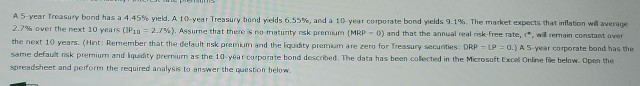What is the yield on this 5-year corporate bond? A 5-year Treasury bond has a 4.45% yield. A 10-yeat Treasury hond yields 6.55%, and a 10-year corporate bond yields 9.1%. The market expects that inflation wilaverage 2.7% over the next 10 years (IP:u= 2.796). Assure that there is no maturity nsk premium (MRP - 0) and that the annual real risk-free rate, 1", will remain constant over the next 10 years. (Hint: Remember that the default nsk premium and the...

• ### Excel Online Structured Activity: Interest rate premiums A5-year Treasury bond has a 4.8 % yield ....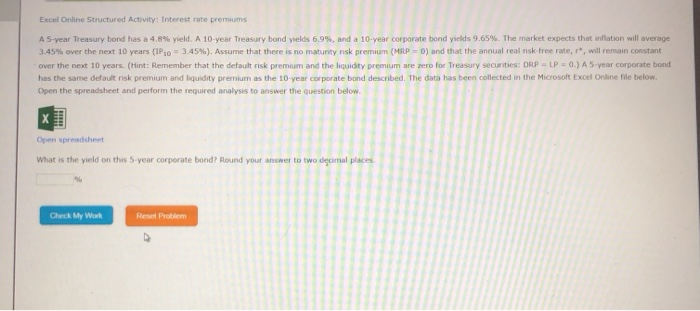Excel Online Structured Activity: Interest rate premiums A5-year Treasury bond has a 4.8 % yield . A 10- year Treasury bond yields 6.9 % , and a 10-year corporate bond yields 9.65 %. The market expects that inflation will average 3.45% over the next 10 years (IP10 3.45 % ) . Assume that there is no maturity nsk premium (MRP 0) and that the annual real risk-free rate, r, will remain constant over the next 10 years. (Hint: Remember that...

• ### LACel Une Juului cu ACLIVILY. Illel CSL lule Pielumns A 5-year Treasury bond has a 3.65%...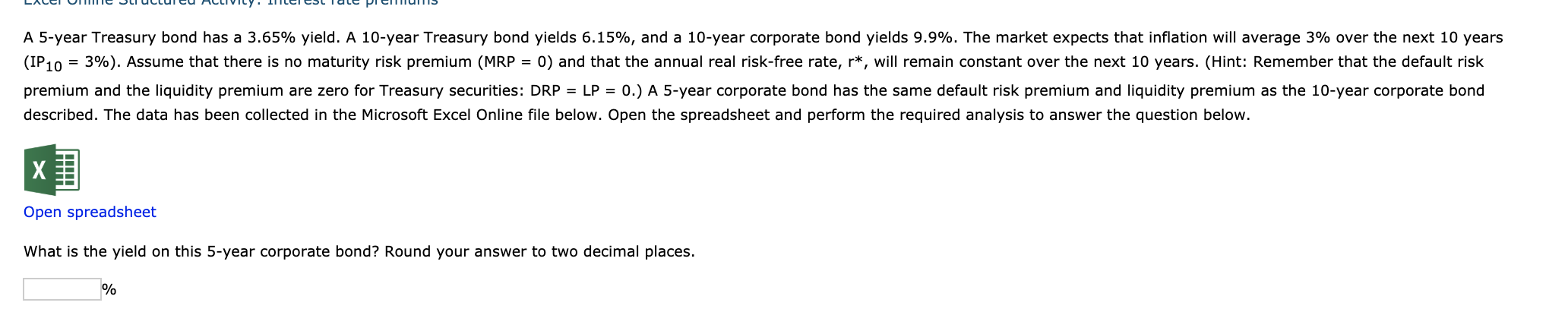LACel Une Juului cu ACLIVILY. Illel CSL lule Pielumns A 5-year Treasury bond has a 3.65% yield. A 10-year Treasury bond yields 6.15%, and a 10-year corporate bond yields 9.9%. The market expects that inflation will average 3% over the next 10 years (IP 10 = 3%). Assume that there is no maturity risk premium (MRP = 0) and that the annual real risk-free rate, r*, will remain constant over the next 10 years. (Hint: Remember that the default risk...

• ### Question 1 Video 0/10 Submit Excel Online Structured Activity: Interest rate premiums A 5-year Treasury bond...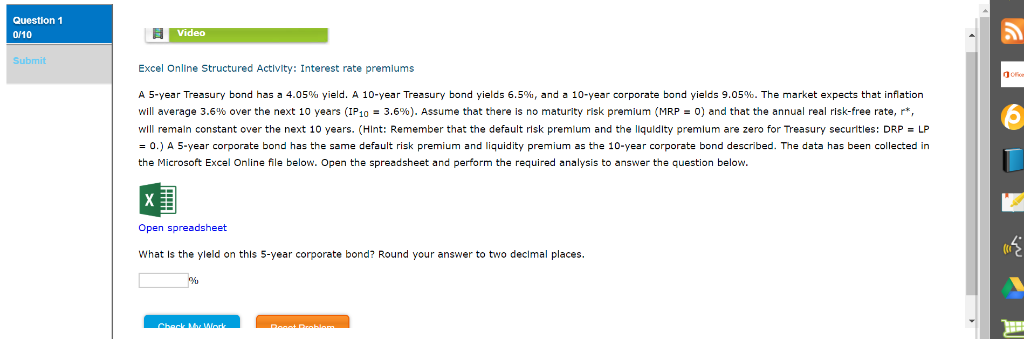Question 1 Video 0/10 Submit Excel Online Structured Activity: Interest rate premiums A 5-year Treasury bond has a 4.05% yield. A 10-year Treasury bond yields 6.5%, and a 10-year corporate bond yields 9.05%. The market expects that infiation no maturity risk premium (MRP = 0) and that the annual real risk-free rate, r* will averaqe 3.6% over the next 10 years (IP10 3.6%). Assume that there wll remaln constant over the next 10 years. (Hint: Remember that the default risk...

• ### Question 1 Video 0/10 Submit Excel Online Structured Activity: Interest rate premiums A 5-year Treasury bond...Question 1 Video 0/10 Submit Excel Online Structured Activity: Interest rate premiums A 5-year Treasury bond has a 4.05% yield. A 10-year Treasury bond yields 6.5%, and a 10-year corporate bond yields 9.05%. The market expects that infiation no maturity risk premium (MRP = 0) and that the annual real risk-free rate, r* will averaqe 3.6% over the next 10 years (IP10 3.6%). Assume that there wll remaln constant over the next 10 years. (Hint: Remember that the default risk...

• ### Video Excel Online Structured Activity: Interest rate premiums A 5-year Treasury band has a 3.5% yield....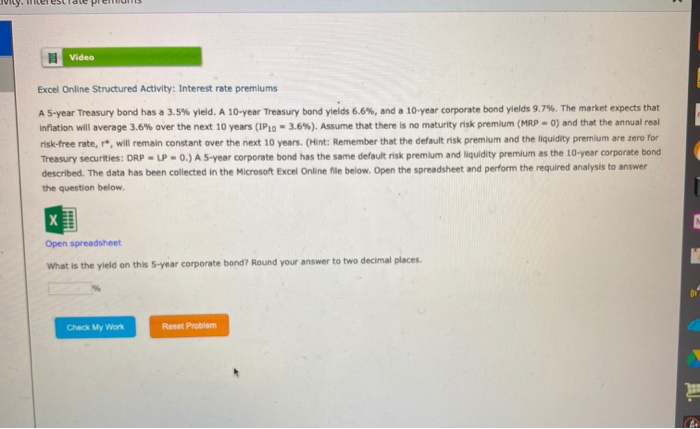Video Excel Online Structured Activity: Interest rate premiums A 5-year Treasury band has a 3.5% yield. A 10-year Treasury bond yields 6.6%, and a 10-year corporate bond yields 9.7%. The market expects that Inflation will average 3.6% over the next 10 years (IP10 -3.6). Assume that there is no maturity risk premium (MRP-0) and that the annual real risk-free rate, , will remain constant over the next 10 years. (Hint: Remember that the default risk premium and the liquidity premium...

• ### ACLIVIly. Interest rate premiums B Video Excel Online Structured Activity: Interest rate premiums A 5-year Treasury...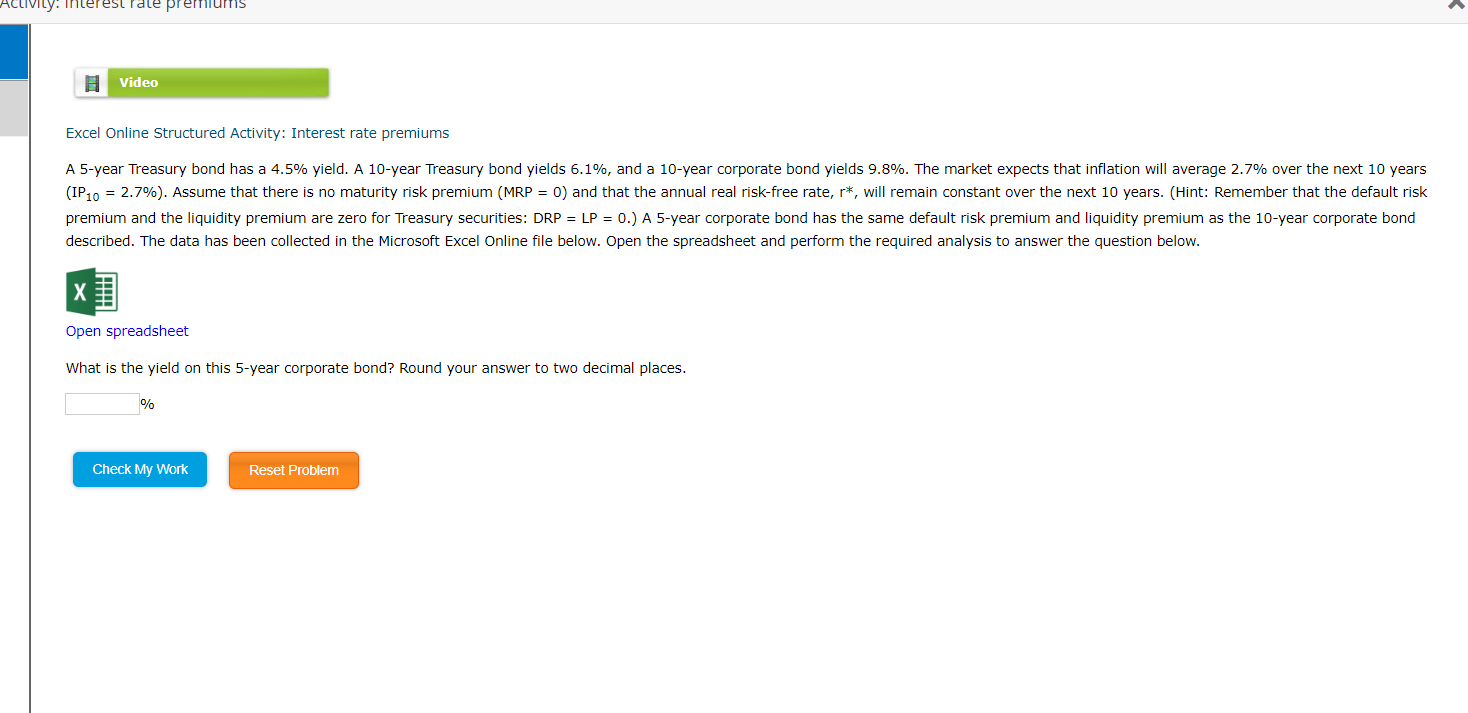ACLIVIly. Interest rate premiums B Video Excel Online Structured Activity: Interest rate premiums A 5-year Treasury bond has a 4.5% yield. A 10-year Treasury bond yields 6.1%, and a 10-year corporate bond yields 9.8%. The market expects that inflation will average 2.7% over the next 10 years (IP 10 = 2.7%). Assume that there is no maturity risk premium (MRP = 0) and that the annual real risk-free rate, r*, will remain constant over the next 10 years. (Hint: Remember...